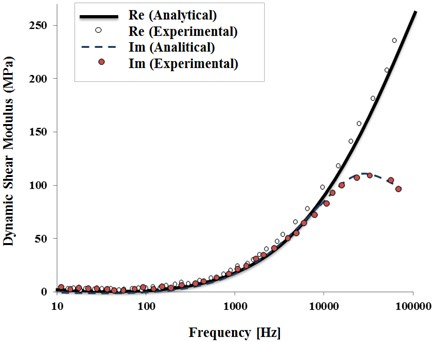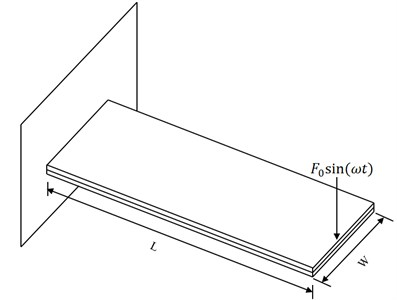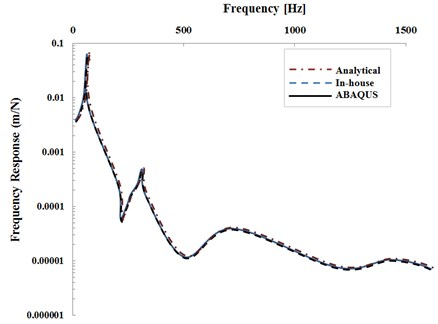Published: 30 December 2015

# Simulation of viscoelastic materials by ABAQUS

1, 2Department of Mechanical Engineering, Parand Branch, Islamic Azad University, Tehran, Iran
Corresponding Author:
Views 336

#### Abstract

The goal of present article is to simulate the behavior of viscoelastic materials. By using ABAQUS software, we can simulate the behavior of viscoelastic materials, conditional to calculate storing shear module and wasting module of viscoelastic materials by the test. First, we give descriptions required parameters such as shear module and loss factor in order to anticipate the behavior of viscoelastic materials. Then, the measurement of each above variable will be illustrated by test. To make use of the result from test, related theories should be explained in brief. Finally, resultant variables are used to simulate in ABAQUS software. The results of test are compared with software results. This comparison indicates that the results are well consistent.

## 1. Introduction

Noise and vibrations are an environmental topic we are always considered to decrease or remove them. In cars, the most common method is adding passive dissipative materials to the body in order to reduce noise and vibration level. Anyway, prediction of noise and vibration is substantial.

Body structures for, e.g., automotive, space, aeronautical and naval applications are, to a large extent, consisting of thin walled structures and corresponding finite element models are most often built from shell elements. Although modelling with three dimensional solid elements is becoming more and more common for thin-walled structures, thanks to the extra-ordinary development of computer hardware capacity during a number of years, engineers will never have unlimited resources in terms of degrees-of-freedom to spend on a finite element model. Therefore, there will always be a tradeoff between the simplicity in modelling using solid modelling and the extra number of degrees-of-freedom it will cause.

It is found from a continuous cooperation over several years with the large and well known car manufacturers, that today, within the automotive industry, shell modelling is the totally dominating finite element modelling (FEM) technique on the car “body-in white” level. Thus, any investigation on the effect of “applied damping material” (ADM) to the structure will have to cope with the fact that the existing base structure FE model is made up of shell elements. Also, almost the whole community of car manufacturers reports large difficulties in how to accurately enough model viscoelastic materials, and to get correlation with measurements. An easy-to-use and accurate material model which can be communicated using only a few parameters is therefore key to an improvement within this field.

One of influential way to reduce vibrational energy is using viscoelastic materials as constrained layer [1, 2]. Loss factor of viscoelastic materials and also the stiffness of constrictive layer attached to the structure are very effective to reduce vibrational energy. Thus, when the main structure is vibrated abundantly, viscoelastic layer is subjected to a huge transformation and its kinetic energy will be converted to thermal.

Several ways have been presented to show the behavior of viscoelastic materials in the ambience of vibration absorbent layers since 1950. One of the ways to simulate the behavior of viscoelastic materials is modeling shear modules by a series of small damping oscillators. According to the same idea, two scientists performed transient and steady state analysis of sandwich panel. The intersection of all recent studies is that parameters in the selected model are accurately known or estimated.

There are two basic information in linear viscoelastic, especially in dynamic behavior of semi-elastics: real and imaginary part of shear module. These variables are severely count on temperature and frequency. Nashif found some information about how to use one-end involved beam for extracting mixed and frequency-depended shear module for different temperatures of a viscoelastic material [3, 4]. The results can be found in Standard ASTM E756-98. The results of these standards have been used in the article .

## 2. Viscoelasticity as reduced fractions

Rubber with nitrile (Acrylic nitrile butadiene) base is one of the most common rubbers. The rubber studied in this example contains 35 % of Black Carbon. Rubber has been stock to the steel plate. In the process of sticking them, temperature and pressure must be high enough; rubber must be also already attached to the steel by a thin layer of glue.

Rubber with nitrile base is basically a viscoelastic and quite incompressible material. Viscoelastic behavior is observed when shear module of material is a function of frequency as Fig. 1. Poisson’s coefficient of this material has been achieved with high accuracy of 0.4993. It has been assumed in this model that strains are little and linear. Thin layer of rubber has been constrained by metal plates which are good thermal conductors. The assumption of being isothermal is also valid for the rubber. Elastic behavior (in isothermal and isotropic conditions) has been modeled by linear viscoelastic model. However, using classic model of viscoelastic materials in which internal variables are first grade according to the variations law, many internal variables and consequently many parameters are needed to describe viscoelastic behavior. The numbers of required parameters are significantly reduced by using reduced fractions. Linear reduced fractions are appropriately consistent with empirical results for a wide range of frequencies and polymers. But this consistency is in single-axis state.

Fig. 1Simple transformations of materialsShear stress equation $\tau \left(t\right)$ can be written as two Couple equations:

1
$\tau \left(t\right)={G}_{0}\gamma \left(t\right)-\left({G}_{0}-{G}_{\infty }\right){\gamma }^{V},{D}^{\alpha }+\frac{1}{{\tau }_{*}^{\alpha }}{\gamma }^{V}=\frac{1}{{\tau }_{*}^{\alpha }}{\gamma }^{V},{\gamma }^{V}=0,$

where $\gamma$ is the (macroscopic) shear while ${\gamma }^{V}$ is an internal variable of shear type representing a distribution of irreversible microstructural processes in the material, ${G}_{0}>\text{0}$ and ${G}_{\mathrm{\infty }}>\text{0}$ are elastic constants which can be identified as instantaneous and longtime shear modulus:

2
${D}^{\alpha }y\left(t\right)=\frac{1}{\mathrm{\Gamma }\left(1-\alpha \right)}\frac{d}{dt}\left[{\int }_{0}^{t}\frac{y\left(s\right)}{\left(t-s{\right)}^{\alpha }}ds\right],$

where ${D}^{\alpha }$ is the fractional derivative operator of order $\alpha \in \left(\text{0, 1}\right)$. Using Fourier transform, operator $D$ is:

3
$F\left[{D}^{\alpha }y\left(t\right)\right]\left(\omega \right)=\left(i\omega {\right)}^{\alpha }F\left[y\left(t\right)\right]\left(\omega \right).$

Using Eqs. (1) and (3), the Eq. (4) will be obtained:

4
$t\left(\omega \right)={G}^{*}\left(\omega \right)\gamma \left(\omega \right),{G}^{*}\left(\omega \right)=\left[{G}_{s}\left(\omega \right)+i{G}_{l}\left(\omega \right)\right]={G}_{0}\frac{{G}_{0}-{G}_{\infty }}{1+\left[{t}_{*}\left(i\omega \right){\right]}^{\alpha }},$

$\mathrm{w}$here ${t}_{\mathrm{*}}$ relaxation constant and ${G}_{\mathrm{*}}$ is denoted the dynamic shear modulus.

Using ASTM E756-98 standard, real and imaginary part of shear module can be obtained . Real and imaginary part of shear module is a function of temperature and frequency shown practically by a one-end involved beam in Fig. 2. A non-contact electromagnetic stimulation has been placed at the free-end of beam. The how to perform the test is presented in ASTM E756-98 . A piezoelectric crystal collects system response at the tingly end. Temperature is increased from –30 to 180 degrees within 10 degrees’ steps. When the sample temperature reached to steady state, all tests will be performed. The required time to achieve desired temperature at each step is about 30 minutes. The length of the beam used for each test is 15 cm and it width 25.4 mm.

Fig. 2The beam used for testShear module, obtained from test results, has two real and imaginary parts. Real and imaginary parts have to be separated. Real and imaginary parts are separated in the Eq. (5):

5
${G}_{0}\frac{\left[1+\mathrm{c}\mathrm{o}\mathrm{s}\left(\frac{\pi \alpha }{2}\right)\right]\left({G}_{0}-{G}_{\mathrm{\infty }}\right)\left(\omega t{\right)}^{\alpha }}{2\left[1+\mathrm{c}\mathrm{o}\mathrm{s}\left(\frac{\pi \alpha }{2}\right)\left(\omega t{\right)}^{\alpha }\right]}+i\frac{\mathrm{s}\mathrm{i}\mathrm{n}\left(\frac{\pi \alpha }{2}\right)\left({G}_{0}-{G}_{\mathrm{\infty }}\right)\left(\omega t{\right)}^{\alpha }}{2\left[1+\mathrm{c}\mathrm{o}\mathrm{s}\left(\frac{\pi \alpha }{2}\right)\left(\omega t{\right)}^{\alpha }\right]}\cdot$

In order to achieve coefficients of the curve easily, it is necessary to define a variable like $B$ instead of $\mathrm{c}\mathrm{o}\mathrm{s}\left(\pi \alpha /2\right)$ in real part of Eq. (5), and then Eq. (6) is given:

6
${G}_{0}\frac{\left[1+B\right]\left({G}_{0}-{G}_{\infty }\right)\left(\omega t{\right)}^{\alpha }}{2\left[1+B\left(\omega t{\right)}^{\alpha }\right]}.$

Fig. 1 shows the results from test. To achieve the parameters of Eq. (6), minimum squares method must be used to have the least error for passing the curve. To do so, MATLAB software was used. Finally, the following values were obtained:

7
${G}_{0}=414\mathrm{}\text{MPa},\mathrm{}\mathrm{}\mathrm{}{G}_{\mathrm{\infty }}=4\mathrm{}\text{MPa},\mathrm{}\mathrm{}\mathrm{}{t}_{\mathrm{*}}=3.31×1{0}^{-6}\mathrm{s},\mathrm{}\mathrm{}\mathrm{}\alpha =\text{0.6.}$

## 3. Viscoelastic materials and ABAQUS

ABAQUS software investigates viscoelastic materials’ behavior in two main fields: time and frequency. In time field, several transformations of these materials are investigated, and in frequency field, the behavior of materials depending on the frequency.

### 3.1. Viscoelastic theory in ABAQUS

Consider a shear test with small strain; this shear strain varies frequently by time:

8
$\gamma \left(t\right)={\gamma }_{0}\mathrm{e}\mathrm{x}\mathrm{p}\left(i\omega t\right).$

It is assumed that this viscoelastic piece has been under oscillation for a long time, and reached to a steady state:

9
$\tau \left(t\right)=\left[{G}_{s}\left(\omega \right)+i{G}_{l}\left(\omega \right)\right]{\gamma }_{0}\mathrm{e}\mathrm{x}\mathrm{p}\left(i\omega t\right).$

This module (mixed) can be dimensionless first by the help of the Eq. (1), and then Fourier transform:

10
$g\left(t\right)=\frac{{G}_{R}\left(t\right)}{{G}_{\mathrm{\infty }}}-1,$

and:

11
${G}_{s}\left(\omega \right)={G}_{\mathrm{\infty }}\left[1-\omega \mathfrak{I}{g}^{\mathrm{*}}\left(\omega \right)\right],\mathrm{}\mathrm{}\mathrm{}{G}_{l}\left(\omega \right)={G}_{\mathrm{\infty }}\left[\omega \mathfrak{R}{g}^{\mathrm{*}}\left(\omega \right)\right].$

When there is a multi-axis stress in the model, ABAQUS assumes that shear module and volume module are independent. The behavior of volume module is defined by two parts of storing volume module ${K}_{s}\left(\omega \right)$ and wasting volume module. A shear module similar to this model’s module can be dimensionless by the help of Fourier transform ${k}^{\mathrm{*}}\left(\omega \right)$ and time-dependent volume module ${K}_{R\left(t\right)}$:

12
${K}_{s}\left(\omega \right)={K}_{\infty }\left[1-\omega \mathfrak{I}{k}^{*}\left(\omega \right)\right],{K}_{l}\left(\omega \right)={K}_{\infty }\left[\omega \mathfrak{R}{k}^{*}\left(\omega \right)\right].$

### 3.2. Defining viscoelastic materials properties in ABAQUS

There are 4 ways to define viscoelastic materials’ properties in frequency field, including:

1 – Determining the coefficients of Peron series;

2 – Results of creeping test;

3 – Results of releasing test;

4 – Determining the frequency-dependent coefficients.

Determining the frequency-dependent coefficients is categorized into two parts of Formula and Tabular methods.

## 4. Relations of formula method

The relations of this part are as follows:

13
${g}^{\mathrm{*}}={g}_{1}^{\mathrm{*}}{f}^{-a},\mathrm{}\mathrm{}\mathrm{}{k}^{\mathrm{*}}={k}_{1}^{\mathrm{*}}{f}^{-a}.$

## 5. Relations of tabular method

The relations of this part are as follows:

14
$\omega \mathfrak{R}{g}^{*}\left(\omega \right)=\frac{{G}_{l}}{{G}_{\infty }},\omega \mathfrak{I}{g}^{*}\left(\omega \right)=1-\frac{{G}_{s}}{{G}_{\infty }},\omega \mathfrak{R}{k}^{*}\left(\omega \right)=\frac{{K}_{l}}{{K}_{\infty }},\omega \mathfrak{I}{k}^{*}\left(\omega \right)=1-\frac{{K}_{s}}{{K}_{\infty }}.$

## 6. Simulation of a real example in ABAQUS software

In this section, we simulate the beam of Fig. 2. In Fig. 2, steel plates are placed in up and down, each of them with thickness of 0.5 mm. They are drawn in Part module of ABAQUS software as Shell. There is viscoelastic material between two mentioned plates, which is made of NBR with thickness of 0.1 mm. In Part module, this part is drawn as Solid.

In Table 1, the properties of elastic section for steel and viscoelastic layer are presented.

In load module, we apply 1 N load on the beam end, and close all freedom degrees at the other end.

Table 1The properties of elastic part

 Poison’s coefficient Yang module (GPa) Density (kg/m3) Steel 0.3 210 7800 Layer NBR 0.4939 0.002757904 1250

## 7. Finding

In the following, the results of test are compared with software results. Fig. 3 indicates that the results are well consistent.

Fig. 3Analytical solution results (dotted mode of answer for tingly support)## 8. Conclusion

By using ABAQUS software, we can simulate the behavior of viscoelastic materials, conditional to calculate storing shear module and wasting module of viscoelastic materials by the test. Table method is the best choice among them. The results of test which are compared with software results indicates that the results are well consistent.